Next: Spring-Coupled Masses Up: Coupled Oscillations Previous: Normal Modes

Normal Coordinates

Since the eigenvectors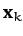, for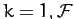, span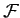-dimensional vector space, we can always write the displacement vector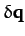as some linear combination of the: i.e.,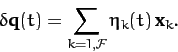(808)

We can regard the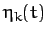as a new set of generalized coordinates, since specifying the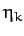is equivalent to specifying the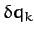(and, hence, the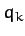). Theare usually termed normal coordinates. According to Equations (799) and (808), the normal coordinates can be written in terms of theas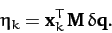(809)

Let us now try to express,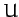, and the equations of motion in terms of the.

The kinetic energy can be written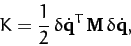(810)

where use has been made of Equation (775). It follows from (808) that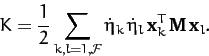(811)

Finally, making use of the orthonormality condition (799), we obtain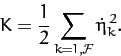(812)

Hence, the kinetic energytakes the form of a diagonal quadratic form when expressed in terms of the normal coordinates.

The potential energy can be written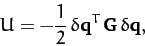(813)

where use has been made of Equations (780). It follows from (808) that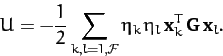(814)

Finally, making use of Equation (788) and the orthonormality condition (799), we obtain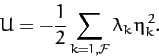(815)

Hence, the potential energyalso takes the form of a diagonal quadratic form when expressed in terms of the normal coordinates.

Writing Lagrange's equations of motion in terms of the normal coordinates, we obtain [cf., Equation (772)]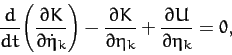(816)

for. Thus, it follows from Equations (812) and (815) that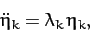(817)

for. In other words, Lagrange's equations reduce to a set ofuncoupled simple harmonic equations when expressed in terms of the normal coordinates. The solutions to the above equations are obvious: i.e.,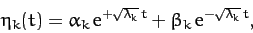(818)

where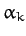and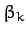are arbitrary constants. Hence, it is clear from Equations (808) and (818) that the most general solution to the perturbed equations of motion is indeed given by Equations (800) and (801).

In conclusion, the equations of motion of a many degree of freedom dynamical system which is slightly perturbed from an equilibrium state take a particularly simple form when expressed in terms of the normal coordinates. Each normal coordinate specifies the instantaneous displacement of an independent mode of oscillation (or secular growth) of the system. Moreover, each normal coordinate oscillates at a characteristic frequency (or grows at a characteristic rate), and is completely unaffected by the other coordinates.Next: Spring-Coupled Masses Up: Coupled Oscillations Previous: Normal Modes
Richard Fitzpatrick 2011-03-31